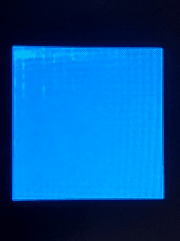# FFT waterfall chart (rain chart)

The FFT waterfall chart is the frequency distribution chart of the data over time. The following will introduce how to use MaixPy to draw the waterfall chart.

## Drawing method

• Prepare time domain signals (such as audio data)
rx = I2S(I2S.DEVICE_0)
rx.set_sample_rate(sample_rate)
audio = rx.record(sample_points)

• Perform FFT operation (use FFT operation on the data and get its frequency distribution)
fft_points = 512
fft_res = FFT.run(audio.to_bytes(),fft_points)
fft_amp = FFT.amplitude(fft_res)

• Draw on image (due to the symmetry of the FFT result, only a part of it needs to be drawn)
hist_x_num = 128
img = image.Image(size=(128,128))
for i in range(hist_x_num):
img[i] = fft_amp[i]


Detailed API reference I2S-API, FFT-API

## Routine

The following example is tested in firmware v0.5.1 MaixDock

Acquire audio data in real time and draw it as an FFT waterfall chart

demo_fft_waterfall.py

effect: